# Review — GPKD: Learning Light-Weight Translation Models from Deep Transformer

## GPKD, Light-Weight Transformerby Knowledge Distillation

Learning Light-Weight Translation Models from Deep Transformer
GPKD, by Northeastern University, and NiuTrans Research
2021 AAAI, Over 10 Citations (Sik-Ho Tsang @ Medium)
Natural Language Processing, NLP, Machine Translation, Transformer, Teacher Student, Knowledge Distillation

• Neural Machine Translation (NMT) system is computationally expensive and memory intensive. A strong but light-weight NMT system is needed.
• A novel group-permutation based knowledge distillation (GPKD) approach is proposed to compressing the deep Transformer model into a shallow model.
• To avoid overfitting, skipping sub-layer method is also proposed.
• The compressed model is 8 times shallower than the deep model, with almost no loss in BLEU.

# Outline

1. Knowledge Distillation (KD) & Sequence-Level KD (SKD)
2. Group-Permutation Based Knowledge Distillation (GPKD)
3. Skipping Sub-Layers for Deep Transformer
4. Experimental Results

# 1. Knowledge Distillation(KD) & Sequence-Level KD (SKD)

• Let FT(x, y) and FS(x, y) represent the predictions of the teacher network and the student network, respectively.
• Then KD can be formulated as follows:
• where L(.) is a loss function to evaluate the distance between FT(x, y) and FS(x, y). (Please feel free to read Distillation if interested.)

The objective can be seen as minimizing the loss function to bridge the gap between the student and its teacher.

• To advance the student model, some prior arts learn from the intermediate layer representations of the teacher network via additional loss functions. However, the additional loss functions require large memory footprint. This is quite challenging when the teacher is extremely deep.
• Alternatively, sequence-level knowledge distillation (SKD) is chosen to simplify the training procedure.

SKD achieves comparable or even higher translation performance than word-level KD method. Concretely, SKD uses the translation results of the teacher network as the gold instead of the ground truth.

In this work, student systems are built upon SKD method.

# 2. Group-Permutation Based Knowledge Distillation (GPKD)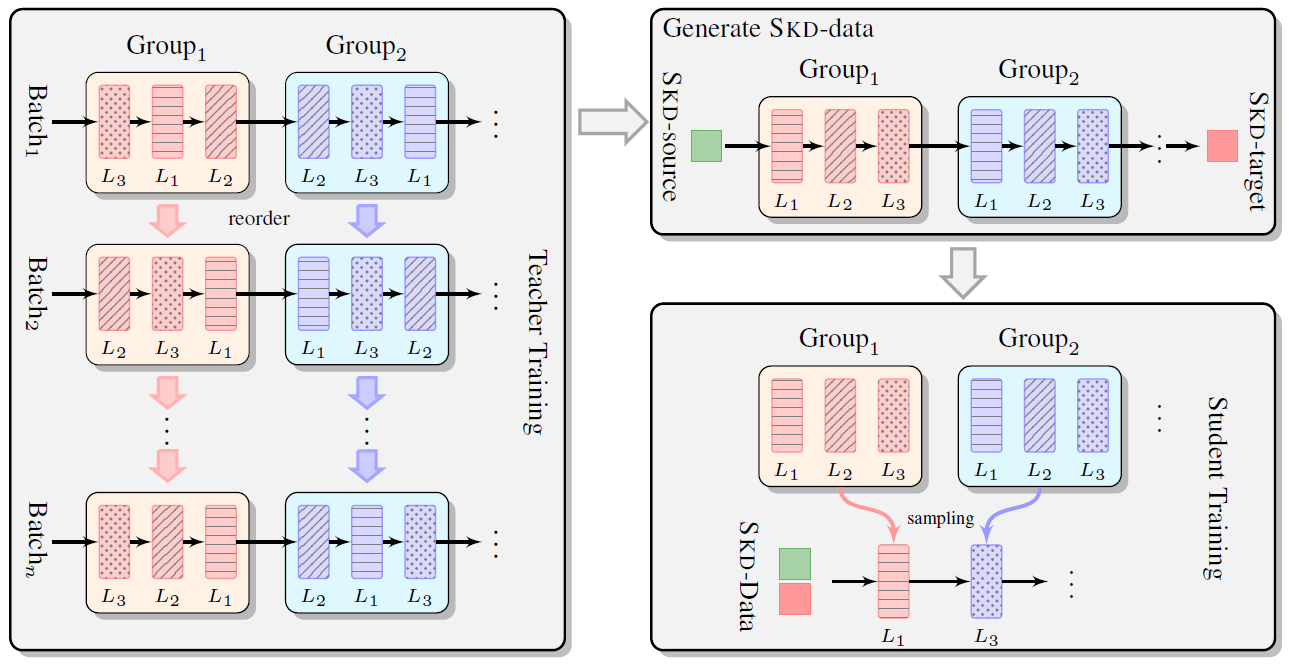An overview of the GPKD method including three stages. Group1 and Group2 correspond to different groups of the stacking layers. L1, L2 and L3 denote the layers in each group.
• The proposed Group-Permutation based Knowledge Distillation (GPKD) method, including three stages:
1. (2.1.): Group-permutation training strategy which rectifies the information flow of the teacher network during training phase.
2. (2.2.): Generate the SKD data through the teacher network.
3. (2.3.): Train the student network with the SKD data. Instead of randomly initializing the parameters of the student network, layers from the teacher network are selected to form the student network, which provides a better initialization.

## 2.1. Group-Permutation Training

• Assuming that the teacher model has N Transformer layers, GPKD aims to extract M layers to form a student model.
• To achieve this goal, the stacking layers of teacher model are first divided into M groups and every adjacent h=N/M layers form a group. The core idea of the proposed method is to make the selected single layer mimic the behavior of its group output.
• Figure Left: Instead of employing additional loss functions to reduce the distance of the intermediate layer representations between the student network and the teacher network, the computation order in each group is simply disturbed when training the teacher network. The layer order is sampled uniformly from all the permutation choices.

## 2.2. Generating SKD Data

• Figure Top Right: Given the training dataset {X, Y}, the teacher network translates the source inputs into the target sentences Z. Then the SKD data is the collection of {X, Z}.

## 2.3. Student Training

• Now, GPKD begins optimizing the student network with the supervision of the teacher.
• One layer from each group is randomly chosen to form a “new” encoder which the encoder depth is reduced from N to M.
• The compression rate is controlled by the hyper-parameter h. One can compress a 48-layer teacher into a 6-layer network by setting h=8, or progressively achieve this goal with h=2 for three times of compression.

## 2.4. DESDAR Architecture

• The GPKD method also fits into the decoder. Hence, a heterogeneous NMT model architecture (AR) is designed, which consists of a deep encoder (DE) and a shallow decoder (SD), abbreviated as (DESDAR).
• Such an architecture can enjoy high translation quality due to the deep encoder and fast inference due to the light decoder. It offers a way of balancing the translation quality and the inference.

# 3. Skipping Sub-Layers for Deep Transformer

## 3.1. Co-Adaptation of Sub-Layers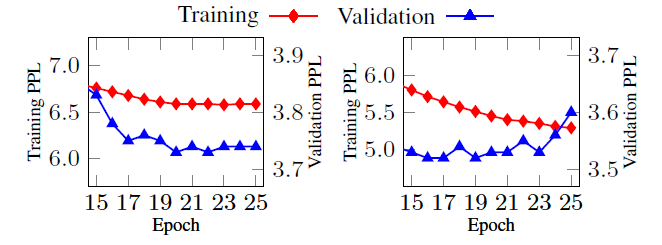Comparison of training and validation PPL on the shallow (left) and the deep (right) models
• Similar to the descriptions by Dropout, there is co-adaptation of the sub-layers. It prevents the model from generalizing well at test time.
• As shown above, clearly, the deep model (48-layer encoder) appears to overfit.

## 3.2. The Skipping Sub-Layer Method

• To address the overfitting problem, either the self-attention sub-layer or the feed-forward sub-layer of the Transformer encoder is dropped for robust training.
• In this paper, both types of the sub-layer follow the Pre-Norm architecture of deep Transformer, i.e. Pre-Norm Transformer:
• where LN() is the layer normalization function, xl is the output of sub-layer l and F() is either the self-attention or feed-forward function.
• A variable M ∈ {0, 1} is used to control how often a sub-layer is omitted.
• where M=0 (or =1) means that the sub-layer is omitted (or reserved).
• The lower-level sub-layers of a deep neural network provide the core representation of the input and the subsequent sub-layers refine that representation. It is natural to skip fewer sub-layers if they are close to the input.
• Let L be the number of layers of the stack and l be the current sub-layer. Then, M is defined as:
• where:
• where Φ=0.4. pl is the rate of omitting the sub-layer.
• For sub-layer l, a variable P is first drawn from the uniform distribution in [0, 1]. Then, M is set to 1 if P>pl, and 0 otherwise.
• Similar to Dropout, for inference, all these sub-models behave like an ensemble model. The output representation of each sub-layer is rescaled by the survival rate 1-pl, like this:
• Then, the final model can make a more accurate prediction by averaging the predictions from 2^(2L) sub-models.

## 3.3. Two-stage Training

• First, the model is trained as usual but early stop it when the model converges on the validation set.
• Then, the Skipping Sub-Layer method is applied to the model and training is continued until the model converges again.

# 4. Experimental Results

## 4.1. The Effect of GPKD Method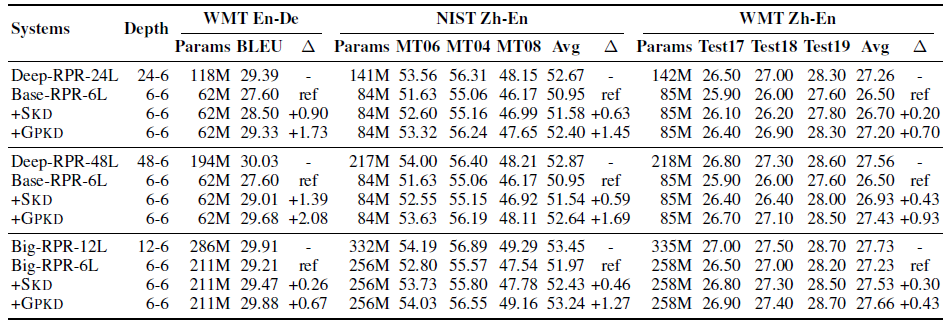The results of the GPKD method applied in encoder side on En-De and Zh-En tasks
• The Relative Position Representation (RPR) from Shaw NAACL’18 is used in the Transformer.
• The hidden sizes of Base and Deep models were 512, and 1024 is for big counterpart.
• 24-layer/48-layer Transformer-Deep systems and 12-layer Transformer-Big systems incorporating the relative position representation (RPR) are successfully trained on three tasks.
• The results are shown above when applying the GPKD method to the encoder side.
• Deep Transformer systems outperform the shallow baselines by a large margin, but the model capacities are 2 or 3 times larger.
• And 6-layer models trained through SKD outperform the shallow baselines by 0.63–1.39 BLEU scores, but there is still a non-negligible gap between them and their deep teachers.

The proposed GPKD method can enable the baselines to perform similarly with the deep teacher systems, and outperforms SKD by 0.41–1.10 BLEU scores on three benchmarks.

Although the compressed systems are 4× or 8× shallower, they only underperform the deep baselines by a small margin.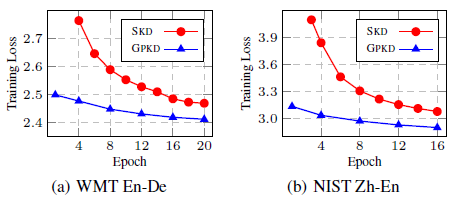The training loss of applying the GPKD (blue) and SKD (red) methods on the WMT En-De, NIST Zh-En tasks, respectively

The above shows that GPKD obtains a much lower training loss than SKD on the WMT En-De and NIST Zh-En, which further verifies the effectiveness of GPKD.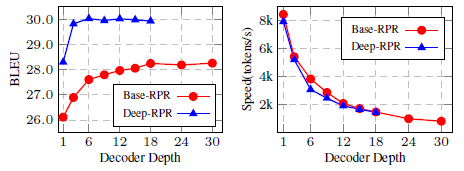BLEU scores [%] and translation speed [tokens/sec] against decoder depth on the En-De task
• The above figure shows that deeper decoders yield modest BLEU improvements but slow down the inference significantly based on a shallow encoder.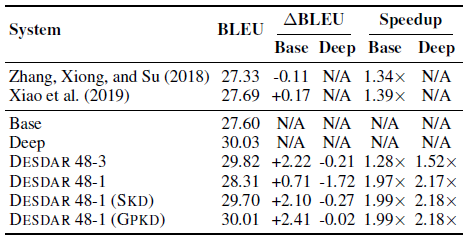BLEU scores [%], inference speedup [×] on the WMT En-De task

DESDAR 48–3 achieves comparable performance with the 48–6 baseline, but speeds up the inference by 1.52×.

• Moreover, GPKD method can enable the DESDAR 48–1 system to perform similarly with the deep baseline, outperforms SKD by nearly +0.31 BLEU scores.
• Interestingly, after knowledge Distillation, the beam search seems like to be not important for the DESDAR systems, which can achieve a 3.2× speedup with no performance sacrifice with the greedy search. This may be due to the fact that the student network learns the soft distribution generated by the teacher network.

## 4.2. The Effect of Skipping Sub-Layer Method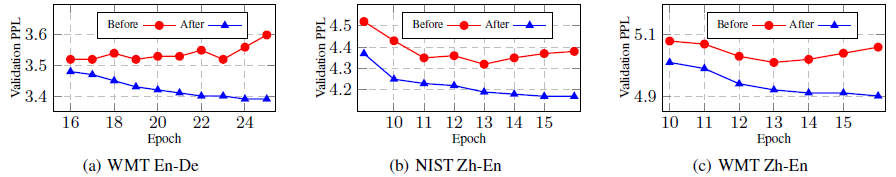The validation PPL of employing the Skipping Sub-Layer method before (red) and after (blue) on En-De, NIST Zh-En and WMT Zh-En tasks, respectively
• Red Line: The 48-layer RPR model converges quickly on three tasks, and the validation PPL goes up later.
• The Skipping Sub-Layer method reduces the overfitting problem and thus achieves a lower PPL (3.39) on the validation set.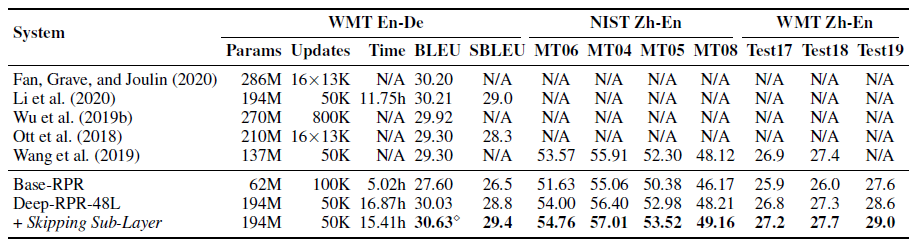BLEU scores [%], parameters and training time on three language pairs

The strong Deep-RPR model trained through the Skipping Sub-Layer approach obtains +0.40–0.72 BLEU improvements on three benchmarks.

## 4.3. SOTA Comparison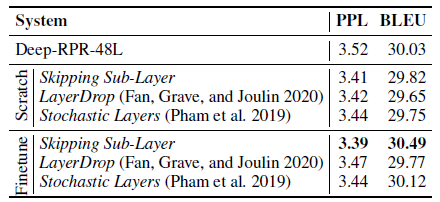Comparison of training from scratch and fine-tune on the WMT En-De task
• All these systems underperform the deep baseline when training them from scratch.

But with fine-tuning, skipping sub-layer performs the best.

## 4.4. Ablation Study

There is no significant difference when skipping FFN or SAN only.

## 4.5. Overall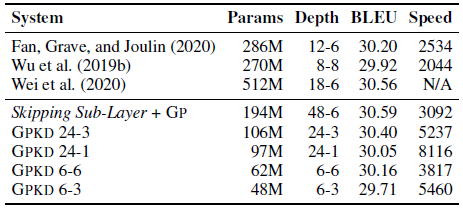The overall results of BLEU scores [%] and translation speed [tokens/sec] on the WMT En-De task
• The above table shows the results of incorporating both the GPKD and Skipping Sub-Layer approaches.
• A 6–6 system achieves comparable performance with the state-of-the-art.

Considering the trade-off between the translation performance and the model storage, one can choose GPKD 6–3 system with satisfactory performance and fast inference speed, or GPKD 24–3 system with both high translation quality and competitive inference speed.

• Another interesting finding here is that shrinking the decoder depth may hurt the BLEU score when the encoder is not strong enough.

## Reference

[2021 AAAI] [GPKD]
Learning Light-Weight Translation Models from Deep Transformer

## Machine Translation

2014 … 2018 [Shaw NAACL’18] 2019 [AdaNorm] [GPT-2] [Pre-Norm Transformer] 2020 [Batch Augment, BA] [GPT-3] [T5] [Pre-LN Transformer] 2021 [ResMLP] [GPKD]

--

--